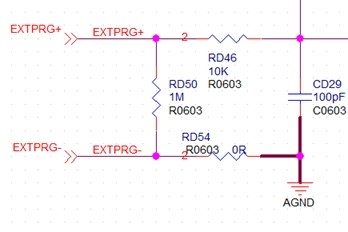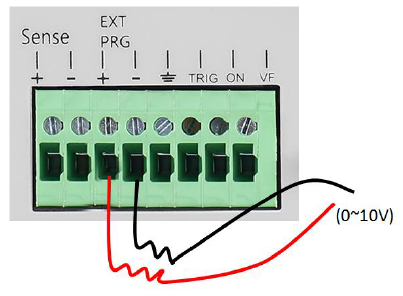# Common SDL1X/X-E performance (not in the data sheet)

June 7, 2019

As with many instruments, there are often details of operation that may not be contained in the manuals or data sheets. To help clarify operations and functions, we created this list to help provide answers to common questions about the SIGLENT SDL1X/X-E models of electronic DC loads:

1. Are the Voltage Sensing inputs (rear panel connector, Sense +, and Sense –) made up as true differential input?

Yes, the Voltage Sense inputs are true different inputs.

2. What is the input resistance (impedance) for each of the “+”and “-” sense inputs?

200 kΩ.

3. Would there be any significant leakage current into the  Voltage Sensing inputs when the output is programmed      to NOT sink any current (current = zero), while the voltage is not zero?

Yes, there is some leakage current. Because the sense channel has a resistance. For example, if the maximum        input voltage is 150 V, the current = 150 V/(200 kΩ*2) = 0.375 mA.

4. What is the permitted voltage range for each of the “+” and  “-” inputs? (For Sense + it must be at least +150 V,        the capability of the instrument?)

150V; The Sense ‘+ ‘and ‘-‘ do not support reverse connections and it also does not have the reverse warning          message.

5. What is the maximum NEGATIVE voltage that can be safely tolerated on the Sense “+” and Sense “-” without     burning the unit?

< 15V.

6. Are the External Programming inputs (rear panel connector, EXT PRG +, and EXT PRG  –) made up as true  differential input?

No, it is not a differential input.   Below is the circuit diagram:7. What is the input resistance (impedance) for each of the “+” and “-” inputs?

The ext prg ‘+’ is 1M ohm and ‘-‘ is 0 ohms (GND).

8. What is the permitted voltage range for each of the “+”and “-” inputs? (It is understood that the maximum differential Programming Input voltage is 10 V)

The maximum Ext prg ‘+’ voltage is 15 V. Ext prg  ‘-‘ is GND. Reverse polarity connections will damage the circuit.

9. What is the maximum NEGATIVE voltage that can be tolerated on the EXT PRG “+” and EXT PRG “-” without burning the unit?

The maximum Ext prg ‘+’ voltage is 15 V.  Ext prg  ‘-‘ is GND. so it can not reverse connection otherwise damage the circuit.

10. Is the continuity to the unit’s GND required for these inputs (for passage of the leakage current from EXT PRG “+” and  EXT PRG “-“, for example), or the programming voltage source should be completely floating with respect to the unit’s GND?

The GND of the programming voltage source must be shared with the input device, same as input -.

11. Can the negative EXT PRG “-” input and Return for the programming voltage source be simply connected to the unit’s GND (rear panel, GND connection right next to the EXT PRG “-”  ?

Yes, EXT PRG’-‘ = GND12. What is the output impedance of the monitoring outputs (rear panel, I Monitor and V Monitor, BNC connectors)?

5 kΩ.